Courses

# Single Correct MCQ Of Electromagnetic Induction And Alternating Current, Past Year Questions JEE Advance, Class 12, Physics

## 16 Questions MCQ Test Class 12 Physics 35 Years JEE Mains &Advance Past year Paper | Single Correct MCQ Of Electromagnetic Induction And Alternating Current, Past Year Questions JEE Advance, Class 12, Physics

Description
This mock test of Single Correct MCQ Of Electromagnetic Induction And Alternating Current, Past Year Questions JEE Advance, Class 12, Physics for JEE helps you for every JEE entrance exam. This contains 16 Multiple Choice Questions for JEE Single Correct MCQ Of Electromagnetic Induction And Alternating Current, Past Year Questions JEE Advance, Class 12, Physics (mcq) to study with solutions a complete question bank. The solved questions answers in this Single Correct MCQ Of Electromagnetic Induction And Alternating Current, Past Year Questions JEE Advance, Class 12, Physics quiz give you a good mix of easy questions and tough questions. JEE students definitely take this Single Correct MCQ Of Electromagnetic Induction And Alternating Current, Past Year Questions JEE Advance, Class 12, Physics exercise for a better result in the exam. You can find other Single Correct MCQ Of Electromagnetic Induction And Alternating Current, Past Year Questions JEE Advance, Class 12, Physics extra questions, long questions & short questions for JEE on EduRev as well by searching above.
QUESTION: 1

### A thin circular ring of area A is held perpendicular to a uniform magnetic field of induction B. A small cut is made in the ring and a galvanometer is connected across the ends such that the total resistance of the circuit is R. When the ring is suddenly squeezed to zero area, the charge flowing through the galvanometer is

Solution:

The current induced will be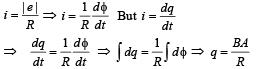QUESTION: 2

### A thin semi-circular conducting ring of radius R is falling with its plane vertical in horizontal magnetic induction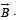At the position MNQ the speed of the ring is v, and the potential difference developed across the ring is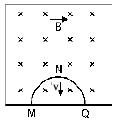Solution:

Induced emf produced across MNQ will be same as the induced emf produced in straight wire MQ.

∴ e = Bvℓ = Bv × 2R with Q at higher potential.

QUESTION: 3

### Two identical circular loops of metal wire are lying on a table without touching each other. Loop-A carries a current which increases with time. In response, the loop-B

Solution:

When the current in the loop A increases, the magnetic lines of force in loop B also increases as loop A is placed near loop B. This induces an emf in B in such a direction that current flows opposite in B (as compared to A). Since currents are in opposite direction, the loop B  is repelled by loop A.QUESTION: 4

A coil of inductance 8.4 mH and resistance 6 Ω is connected to a 12 V battery. The current in the coil is 1.0 A at approximately the time

Solution: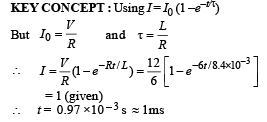QUESTION: 5

A uniform but time-varying magnetic field B(t) exists in a circular region of radius a and is directed into the plane of the paper, as shown. The magnitude of the induced electric field at point P at a distance r from the centre of the circular region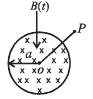Solution: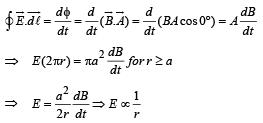QUESTION: 6

A coil of wire having inductance and resistance has a conducting ring placed coaxially within it. The coil is connected to a battery at time t = 0, so that a time-dependent current l1(t) starts flowing through the coil. If I2(t) is the current induced in the ring, and B(t) is the magnetic field at the axis of the coil due to I1(t), then as a function of time (t > 0), the product I2(t) B(t)

Solution:

KEY CONCEPT : The magnetic field at the centre of the coil

B(t) = µ0nI1.

As the current increases, B will also increase with time till it reaches a maximum value (when the current becomes steady).
The induced emf in the ring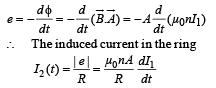[NOTE :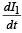decreases with time and hence I2 alsodecreases with time.] Where  I1 = Imax (1 – e –t/t)
The relevant graphs are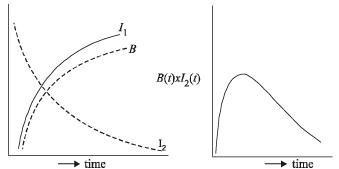QUESTION: 7

A metallic square loop ABCD is moving in its own plane with velocity v in a uniform magnetic field perpendicular to its plane as shown in the figure. An electric field is induced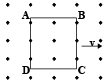Solution:

NOTE : Electric field will be induced, as ABCD moves, in both AD and BC. The metallic square loop moves in its own plane with velocity v. A uniform magnetic field is imposed perpendicular to the plane of the square loop. AD and BC are perpendicular to the velocity as well as perpendicular to applied.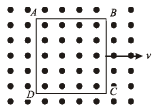QUESTION: 8

Two circular coils can be arranged in any of the three situations shown in the figure. Their mutual inductance will be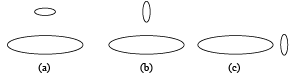Solution:

Clearly the flux linkage is maximum in case (a) due to the spatial arrangement of the two loops.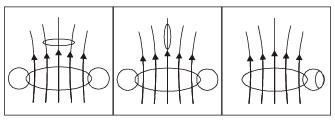QUESTION: 9

As shown in the figure, P and Q are two coaxial conducting loops separated by some distance. When the switch S is closed, a clockwise current IP flows in P (as seen by E) and an induced current IQ1 flows in Q. The switch remains closed for a long time. When S is opened, a current IQ2 flows in Q.
Then the direction IQ1 and IQ2 (as seen by E) are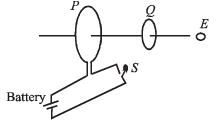Solution:

When switch S is closed, a magnetic field is set-up in the space around P. The field lines threading Q increases in the direction from right to left. According to Lenz's law, IQ1 will flow so as to oppose the cause and flow in anticlockwise direction as seen by E.
Reverse is the case when S is opened. IQ2 will be clockwise.

QUESTION: 10

A short-circuited coil is placed in a time-varying magnetic field. Electrical power is dissipated due to the current induced in the coil. If the number of turns were to be quadrupled and the wire radius halved, the electrical power dissipated would be

Solution:

KEY CONCEPT :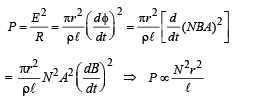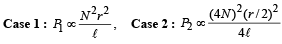NOTE : When we decrease the radius of the wire, its length increases but volume remains the same]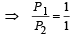∴ Power remains the same.

QUESTION: 11

When an AC source of emf e = E0 sin(100t) is connected across a circuit, the phase difference between the emf e and the current i in the circuit is observed to be π/4 , as shown in the diagram. If the circuit consists possibly only of R-C or R-L or L-C in series, find the relationship between the two elements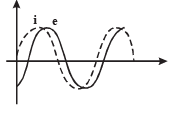Solution:

NOTE :  Since current leads emf (as seen from the graph), therefore, this is an R – C circuit.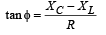Here φ = 45°

∴ XC = R [XL = 0 as there is no inductor]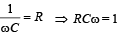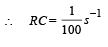QUESTION: 12

A small bar magnet is being slowly inserted with constant velocity inside a solenoid as shown in figure. Which graph best represents the relationship between emf induced with time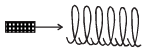Solution:

KEY CONCEPT : Initially, φB increases as magnet approaches the solenoid

∴ ε = – ve and increasing in magnitude. When magnet is moving inside the solenoid, increase in φB slow down and finally φB starts decreasing

∴ emf is positive and increasing.
Only graph (c) shows these characteristic.

QUESTION: 13

An infinitely long cylinder is kept parallel to an uniform magnetic field B directed along positive z-axis. The direction of induced current as seen from the z-axis will be

Solution:

KEY CONCEPT : For a current to induce in cylindrical conducting rod,

(a) the conducting rod should cut magnetic lines of force which will happen only when the conducting rod is moving. Since conducting rod is  at rest, no current will be induced.
(b) the magnitude/direction of the magnetic field changes.
A changing magnetic field will create an electric field which can apply force on the free electrons of the conducting rod and a current will get induced.
But since the magnetic field is constant, no current will be induced.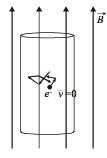QUESTION: 14

Find the time constant (in μs) for the given RC circuits in the given order respectively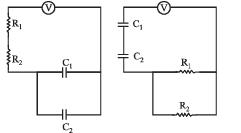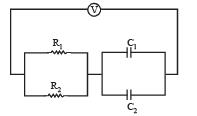R1 = 1W, R2 = 2W, C1 = 4μF , C2 =2μF

Solution:

KEY CONCEPT :

Time constant of  R – C circuit is τ = Req Ceq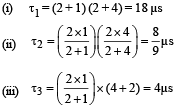QUESTION: 15

The figure shows certain wire segments joined together to form a coplanar loop. The loop is placed in a perpendicular magnetic field in the direction going into the plane of the figure. The magnitude of the field increases with time. I1 and I2 are the currents in the segments ab and cd. Then,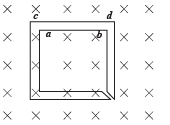Solution:

The magnetic field is increasing in the downward direction. Therefore, according to Lenz’s law the current I1 will flow in the direction ab and I2 in the direction dc.

QUESTION: 16

An AC voltage source of variable angular frequency ω and fixed amplitude V0 is connected in series with a capacitance C and an electric bulb of resistance R (inductance zero). When ω is increased

Solution: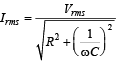As ω increases, Irms increases.

Therefore the bulb glows brighter